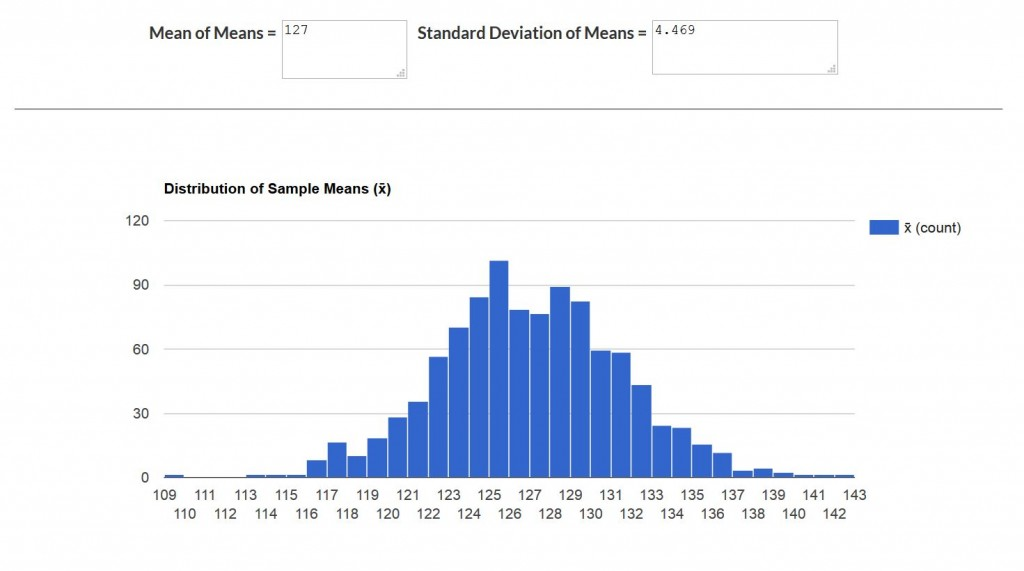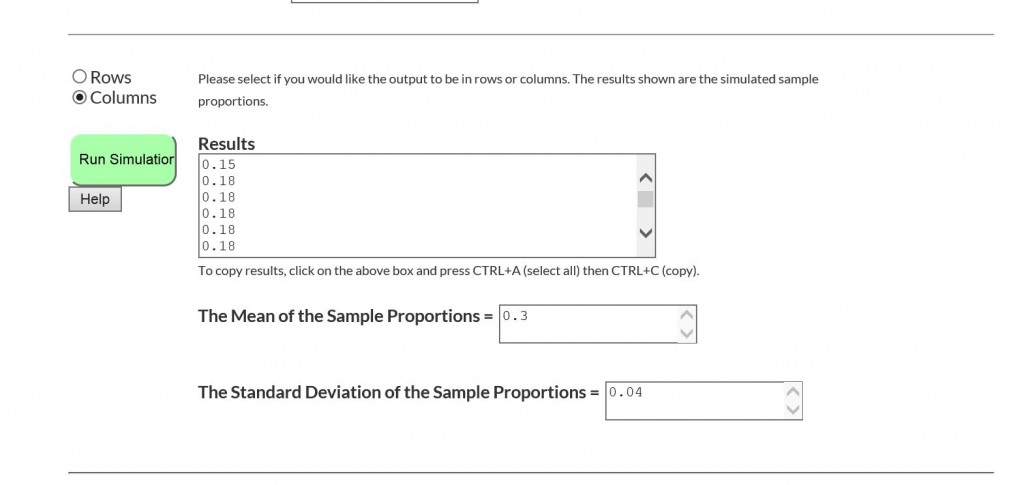Posted on

# New Functionality in Statistical Simulators – CC Alg II – by Kirk

Recently my good friend Joey Shavelle of Jolo Studios put some additional functionality into two of our statistical simulators. For the sample mean simulator he added code that calculates the mean of the means and the standard deviation of the means. Here’s what it looks like when I had a population mean of 127, a standard deviation of 32, and a sample size of 50 with 1000 simulations:(Click on the image to see it more clearly.)

He also added the same code to the sample proportion simulator that calculated the mean sample proportion and the standard deviation of the sample proportions. Here’s a shot of what it looked like when the population p was 0.3, a sample of size 100 was used, with 1000 simulations:So, check them out and use this new functionality in our lessons that require it if you haven’t already finished statistics at this point.